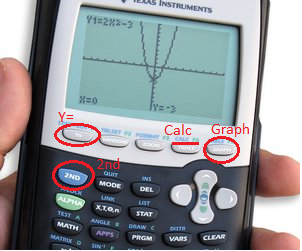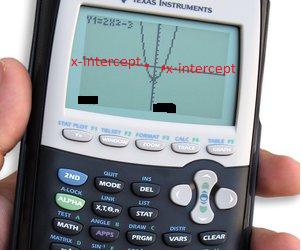# Finding Zeros of Functions & Polynomials on a Graphing Calculator

Instructor: David Karsner

David holds a Master of Arts in Education

When a function or polynomial is graphed on a x,y coordinate grid, it could possibly cross the x-axis. The point(s) at which the graph and the x-axis intersect are called zeros. Graphing calculators have functions that allow you to find the locations of these points if they exist.

## What Does Finding the Zero Mean?

Imagine waking up one morning at 7am and the thermometer reads -3 degrees. Later that day at 5pm it reads 12 degrees. Can you use logic to assume that at some point during the day the thermometer read zero degrees? Well of course you can.

It's that same type of logic that allows a graphing calculator to find the zeros of a function/polynomial. Those zeros are the points in which the function moves from being negative to positive.

If you have a function with two variables then you can graph the solutions onto a x,y coordinate grid. The point(s) on the graph that are of interest in this lesson are the points that cross the x-axis. This point goes by several names: x-intercept, root, and zero. To find the zero of a function means to determine the x value that when inputted into the function will yield the y value of zero. Keep in mind that a function may have none, one, or more zeros.

## When is the Calculator Necessary?

When the function is a lower order polynomial such as a linear or quadratic, a graphing calculator is not necessary. The zeros of these functions be easily found without one. For example, f(x) = 2x+1 is a linear function. You can find the zero of this function by substituting f(x) with 0 and then solving for x.

2x + 1 = 0 subtract the 1,

2x = -1, divide by 2,

x = -1/2.

-1/2 would be the zero of this function.

When the functions are not lower order polynomials then the process is greatly aided by the use of a graphing calculator.

## How to Use a Graphing Calculator to Find the Zero

Note: There are many graphing calculators out there but the most common one is the TI-83, and TI-84 models, so we will refer to those. If you have another type, you may have to select different buttons.

The three buttons you will need to use are:

• the 'Y=',
• the 'GRAPH', and
• the 'CALC'.

Take a moment to find where these buttons are. The 'Y=' button is the top left button on the calculator. The 'GRAPH' button is the top right on the calculator. The 'CALC' button shares the button with the 'TRACE' button, which is the second one from the right in the top row.

Notice how the 'CALC' button is written above the button in a different color. This means that it is a second function button. To access 'CALC' you should select the 2ND button and then select 'TRACE/CALC' button.### Step One: Entering the Function.

This step requires the use of the 'Y=' button. Once that button is selected it will show on the screen several 'Y='s with different subscripts. If there is already something after the equal sign in any of the 'Y='s then clear them out. Be sure that your function/polynomial is solved for y and enter the function into the calculator.

### Step Two: Graphing the Function.

This is done by selecting the 'GRAPH' button. You will need to make sure that the window that the calculator is showing is the correct size. If you cannot see the point(s) where the graph intersects the x-axis; adjust the window until you do see it. You can adjust the window by selecting 'ZOOM' or 'WINDOW' buttons on the top row.### Step Three: Calculating the Zero.

You are now telling your calculator to find the x at which the y value goes from negative to positive.

• You should select the 'CALC' button which requires the 2ND button first. It will give you a list of options.
• Choose zero (on some calculators this will say 'root'). The calculator will go back to the graph screen and the have the words 'Left Bound'.
• Move the cursor to a point that is left of the x intercept that you are interested in. Push 'ENTER'. It will then say 'Right Bound'.
• Move the cursor to the right of the same x-intercept and push 'ENTER'.
• It will then say 'Guess', hit 'ENTER' again.

To unlock this lesson you must be a Study.com Member.

### Register to view this lesson

Are you a student or a teacher?